# Chapter 4 algorithmic efficiency handouts (with notes)

mailund
12 de Nov de 2018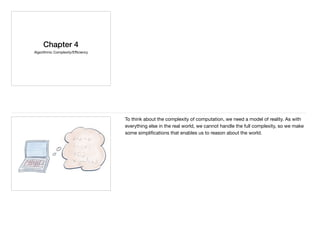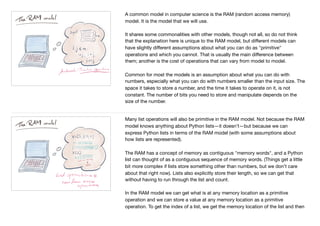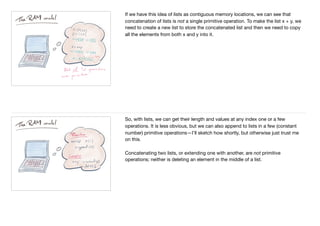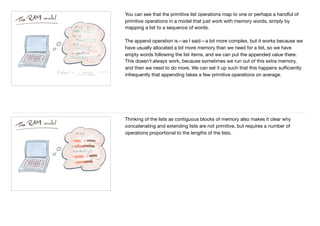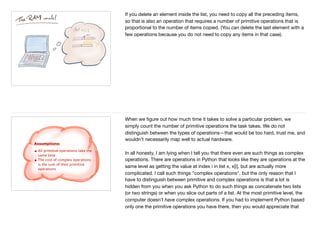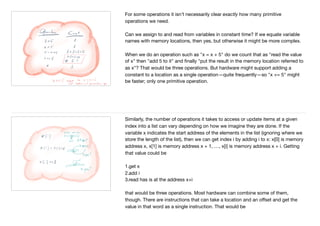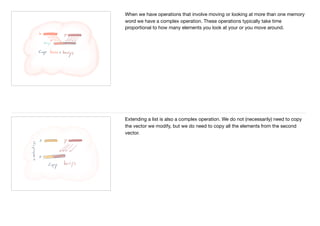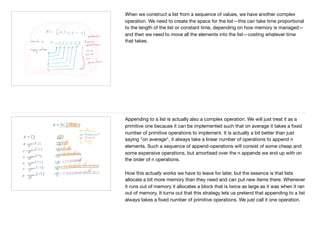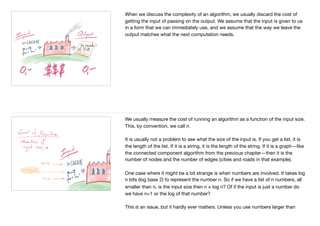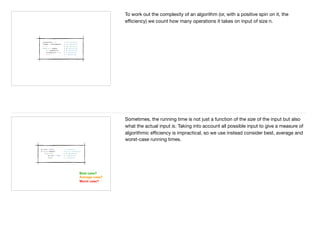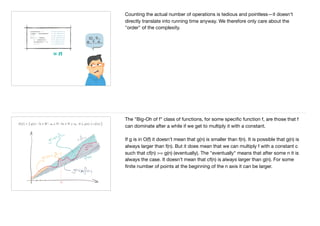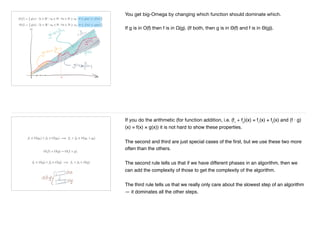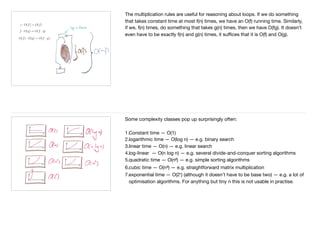1 de 14

### Chapter 4 algorithmic efficiency handouts (with notes)

1. Chapter 4 Algorithmic Complexity/Eﬃciency To think about the complexity of computation, we need a model of reality. As with everything else in the real world, we cannot handle the full complexity, so we make some simpliﬁcations that enables us to reason about the world.
2. A common model in computer science is the RAM (random access memory) model. It is the model that we will use. It shares some commonalities with other models, though not all, so do not think that the explanation here is unique to the RAM model, but diﬀerent models can have slightly diﬀerent assumptions about what you can do as "primitive" operations and which you cannot. That is usually the main diﬀerence between them; another is the cost of operations that can vary from model to model. Common for most the models is an assumption about what you can do with numbers, especially what you can do with numbers smaller than the input size. The space it takes to store a number, and the time it takes to operate on it, is not constant. The number of bits you need to store and manipulate depends on the size of the number. Many list operations will also be primitive in the RAM model. Not because the RAM model knows anything about Python lists—it doesn’t—but because we can express Python lists in terms of the RAM model (with some assumptions about how lists are represented). The RAM has a concept of memory as contiguous "memory words", and a Python list can thought of as a contiguous sequence of memory words. (Things get a little bit more complex if lists store something other than numbers, but we don’t care about that right now). Lists also explicitly store their length, so we can get that without having to run through the list and count. In the RAM model we can get what is at any memory location as a primitive operation and we can store a value at any memory location as a primitive operation. To get the index of a list, we get the memory location of the list and then
3. If we have this idea of lists as contiguous memory locations, we can see that concatenation of lists is not a single primitive operation. To make the list x + y, we need to create a new list to store the concatenated list and then we need to copy all the elements from both x and y into it. So, with lists, we can get their length and values at any index one or a few operations. It is less obvious, but we can also append to lists in a few (constant number) primitive operations—I’ll sketch how shortly, but otherwise just trust me on this. Concatenating two lists, or extending one with another, are not primitive operations; neither is deleting an element in the middle of a list.
4. You can see that the primitive list operations map to one or perhaps a handful of primitive operations in a model that just work with memory words, simply by mapping a list to a sequence of words. The append operation is—as I said—a bit more complex, but it works because we have usually allocated a bit more memory than we need for a list, so we have empty words following the list items, and we can put the appended value there. This doesn’t always work, because sometimes we run out of this extra memory, and then we need to do more. We can set it up such that this happens suﬃciently infrequently that appending takes a few primitive operations on average. Thinking of the lists as contiguous blocks of memory also makes it clear why concatenating and extending lists are not primitive, but requires a number of operations proportional to the lengths of the lists.
5. If you delete an element inside the list, you need to copy all the preceding items, so that is also an operation that requires a number of primitive operations that is proportional to the number of items copied. (You can delete the last element with a few operations because you do not need to copy any items in that case). Assumptions: • All primitive operations take the same time • The cost of complex operations is the sum of their primitive operations When we ﬁgure out how much time it takes to solve a particular problem, we simply count the number of primitive operations the task takes. We do not distinguish between the types of operations—that would be too hard, trust me, and wouldn’t necessarily map well to actual hardware. In all honesty, I am lying when I tell you that there even are such things as complex operations. There are operations in Python that looks like they are operations at the same level as getting the value at index i in list x, x[i], but are actually more complicated. I call such things "complex operations", but the only reason that I have to distinguish between primitive and complex operations is that a lot is hidden from you when you ask Python to do such things as concatenate two lists (or two strings) or when you slice out parts of a list. At the most primitive level, the computer doesn’t have complex operations. If you had to implement Python based only one the primitive operations you have there, then you would appreciate that